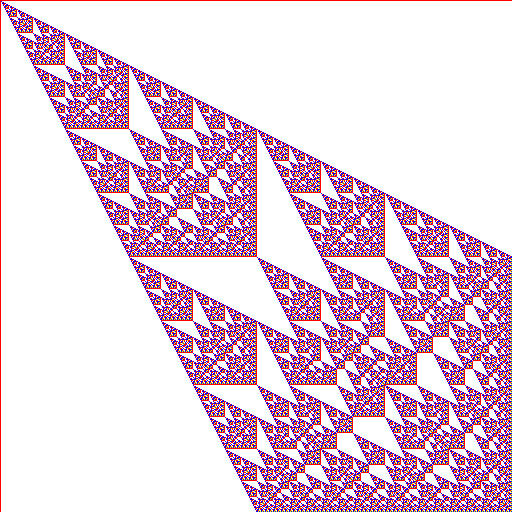## Single Bat

### Info

Denote the elements of the field $F_4$ by $\{0, 1, w, w + 1\}$, where $w$ satisfies the following equation with coefficients in $F_2: w^2 + w + 1 = 0$. Single Bat is a recurrent double sequence defined by $a(i, 0) = a(0, j) = 1$ and $a(i, j) = f(a(i, j-1), a(i-1, j-1), a(i-1, j))$, where $f(x, y, z) = x + (w + 1) x^2 + w y^2 + z + (w + 1) z^2$. This recurrent double sequence can be also obtained using a system of substitutions of type 4 -> 8 with 43 rules.

The system of substitutions is too large to be presented here.

### Patch